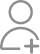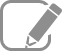Learn Math Olympiad from the Best Tutors

•Affordable fees
•1-1 or Group class
•Flexible Timings
•Verified Tutors

Search in

# If the word ASSASSINATION is put in a box and a word is drawn at random. What is the: 1) Probability of getting a letter A, 2) Probability of getting a letter S.Follow 15AnswerMaths Tutor

1)3/13,2)4/13Tutor

First of all the question should read " a letter" is drawn rather than "a word" is drawn. Now probability of any event E is given by P(E) = n(E)/n(S). where n(E) represents the number of elements of even E and n(S) represents the number of elements in the sample space S. Thus, Answer to Question (1)...
First of all the question should read " a letter" is drawn rather than "a word" is drawn. Now probability of any event E is given by P(E) = n(E)/n(S). where n(E) represents the number of elements of even E and n(S) represents the number of elements in the sample space S. Thus, Answer to Question (1) is 3/13 as n(S) = number of letters in ASSASSINATION= 13 and n(E) = number of A's in ASSASSINATION = 3. Similarly, answer to question (2) is 4/13 as n(S) =13 and n(E) = number of S's = 4. read lessTutor

1) 3/13 AND 2) 4/13

1). Total number of A's in the word are 3. Total letters in the word are 13. Therefore, P(A)= 3/13. 2). Total number of S's in the given word are 4. Therefore, P(S)= 4/13

Total letters=13 Total A's=3 Total S's = 4 Probability for A =No. of A/ Total letters = 3/13 Probability for S= No. Of S/Total letters = 4/13Engineer, Mathamatician, Singer

First of all look at the word and find out howmany A and S we have in the word ASSASSINATION. We have 3 A and 4 S. Therefore 1) the probability of picking up A is 3/13 where 13 is the total number of letters in the word. 2) the probability of picking up S is 4/13.Tutor

Ans is 3/13.total no of A is 3 and total letter are there is 13 so according to rule probability to getting letter A is 3/13Maths Tutor

Probability of getting A is 3/13 and probability of getting s is 4/13Tutor

Answer will be 3/13 and 4/13 As we have Total: 13 letters Out of which we have 3 'A' and 4 'B'.

This word has 13 letters in which 6 distinct types are there. AAA, SSSS, II, NN, T, O. 1) A can be picked 3 times. Probability= 3/13 2) S can be picked 4 times. Probability=4/13

View 14 more Answers

Related Questions03/06/2018
2604/05/2018
42

Now ask question in any of the 1000+ Categories, and get Answers from Tutors and Trainers on UrbanPro.com

Related Lessons

Methods to Solve a Functional Equations
A function is a mapping between a set of inputs and a set of outputs with the property that eachInput is related to. In other words, a function is a mapping between two sets A and B.Tips to Crack Maths Olympiad IMO Class 1-7
Mathematics Olympiad is a quite interesting way to test one’s mathematical prowess and further explore the universe of number tricks and crunching. It is also a forever regular debate among math...Logarithm with JEE Example
JEE 2011 Question on Logarithm Let (x0,y0) be the solution of the following equations: (2x)ln2 = (3y)ln3 3lnx = 2lnyThen x0 is(A)1 6(B)1 3(C)1 2(D) 6Answer and Comments: (C). We can treat the data as...Find Math Olympiad classes near you

Looking for Math Olympiad classes?

Learn from the Best Tutors on UrbanPro

Are you a Tutor or Training Institute?

Join UrbanPro Today to find students near you
X

### Looking for Math Olympiad Classes?

The best tutors for Math Olympiad Classes are on UrbanPro

• Select the best Tutor
• Book & Attend a Free Demo
• Pay and start Learning### Learn Math Olympiad with the Best Tutors

The best Tutors for Math Olympiad Classes are on UrbanProUrbanPro.com is India's largest network of most trusted tutors and institutes. Over 55 lakh students rely on UrbanPro.com, to fulfill their learning requirements across 1,000+ categories. Using UrbanPro.com, parents, and students can compare multiple Tutors and Institutes and choose the one that best suits their requirements. More than 7.5 lakh verified Tutors and Institutes are helping millions of students every day and growing their tutoring business on UrbanPro.com. Whether you are looking for a tutor to learn mathematics, a German language trainer to brush up your German language skills or an institute to upgrade your IT skills, we have got the best selection of Tutors and Training Institutes for you. Read more